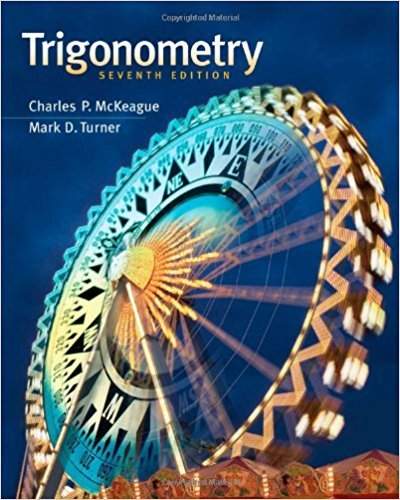×
Log in to StudySoup
Get Full Access to Trigonometry - Textbook Survival Guide
Join StudySoup for FREE
Get Full Access to Trigonometry - Textbook Survival Guide

Already have an account? Login here
×
Reset your password

# Solutions for Chapter 1.5: More on Identities## Full solutions for Trigonometry | 7th Edition

ISBN: 9781111826857Solutions for Chapter 1.5: More on Identities

Solutions for Chapter 1.5
4 5 0 360 Reviews
11
2
##### ISBN: 9781111826857

This textbook survival guide was created for the textbook: Trigonometry, edition: 7. This expansive textbook survival guide covers the following chapters and their solutions. Chapter 1.5: More on Identities includes 109 full step-by-step solutions. Trigonometry was written by and is associated to the ISBN: 9781111826857. Since 109 problems in chapter 1.5: More on Identities have been answered, more than 51531 students have viewed full step-by-step solutions from this chapter.

Key Math Terms and definitions covered in this textbook
• Adjacency matrix of a graph.

Square matrix with aij = 1 when there is an edge from node i to node j; otherwise aij = O. A = AT when edges go both ways (undirected). Adjacency matrix of a graph. Square matrix with aij = 1 when there is an edge from node i to node j; otherwise aij = O. A = AT when edges go both ways (undirected).

• Cholesky factorization

A = CTC = (L.J]))(L.J]))T for positive definite A.

• Cofactor Cij.

Remove row i and column j; multiply the determinant by (-I)i + j •

• Complex conjugate

z = a - ib for any complex number z = a + ib. Then zz = Iz12.

• Echelon matrix U.

The first nonzero entry (the pivot) in each row comes in a later column than the pivot in the previous row. All zero rows come last.

• Elimination matrix = Elementary matrix Eij.

The identity matrix with an extra -eij in the i, j entry (i #- j). Then Eij A subtracts eij times row j of A from row i.

• Ellipse (or ellipsoid) x T Ax = 1.

A must be positive definite; the axes of the ellipse are eigenvectors of A, with lengths 1/.JI. (For IIx II = 1 the vectors y = Ax lie on the ellipse IIA-1 yll2 = Y T(AAT)-1 Y = 1 displayed by eigshow; axis lengths ad

• Fourier matrix F.

Entries Fjk = e21Cijk/n give orthogonal columns FT F = nI. Then y = Fe is the (inverse) Discrete Fourier Transform Y j = L cke21Cijk/n.

• Full row rank r = m.

Independent rows, at least one solution to Ax = b, column space is all of Rm. Full rank means full column rank or full row rank.

• Iterative method.

A sequence of steps intended to approach the desired solution.

• Left inverse A+.

If A has full column rank n, then A+ = (AT A)-I AT has A+ A = In.

• Pascal matrix

Ps = pascal(n) = the symmetric matrix with binomial entries (i1~;2). Ps = PL Pu all contain Pascal's triangle with det = 1 (see Pascal in the index).

• Rank one matrix A = uvT f=. O.

Column and row spaces = lines cu and cv.

• Right inverse A+.

If A has full row rank m, then A+ = AT(AAT)-l has AA+ = 1m.

• Rotation matrix

R = [~ CS ] rotates the plane by () and R- 1 = RT rotates back by -(). Eigenvalues are eiO and e-iO , eigenvectors are (1, ±i). c, s = cos (), sin ().

• Schwarz inequality

Iv·wl < IIvll IIwll.Then IvTAwl2 < (vT Av)(wT Aw) for pos def A.

• Toeplitz matrix.

Constant down each diagonal = time-invariant (shift-invariant) filter.

• Unitary matrix UH = U T = U-I.

Orthonormal columns (complex analog of Q).

• Vandermonde matrix V.

V c = b gives coefficients of p(x) = Co + ... + Cn_IXn- 1 with P(Xi) = bi. Vij = (Xi)j-I and det V = product of (Xk - Xi) for k > i.

• Wavelets Wjk(t).

Stretch and shift the time axis to create Wjk(t) = woo(2j t - k).